# NCERT Solutions for Class 7 Maths Chapter 11 Perimeter and Area

NCERT Solutions for Class 7 Maths Chapter 11 Perimeter and Area: If you want to cover the floor of the room. The first question that comes in mind is how many square meters of flooring you need? It can be done by measuring the area of the room. In the practical case, you will buy slightly higher than the required area since there may be cuts and joints required for the flooring. In CBSE NCERT solutions for class 7 maths chapter 11 perimeter and area, you will get questions related to applications of perimeter and area. Perimeter is the distance around a closed figure and the area is the space occupied by a close figure. The perimeter can be calculated by adding the length of all sides of the closed figure. In this chapter, you will study the perimeters of some simple geometry like rectangle, triangle, parallelogram, and circles. Also, you will learn to calculate the area of rectangle, triangle, parallelogram, and circles. In solutions of NCERT for class 7 maths chapter 11 perimeter and area, you will get all questions related to calculating the area and perimeter only. You should try to solve all the problems including examples for a better understanding of the concepts. Before solving the problems, you must convert all the given values to a common unit so you can get rid of the confusion. If you are given a complex geometry to calculate the area, you can divide it into smaller parts and the total area would be the sum of the area of all the parts. In CBSE NCERT solutions for class 7 maths chapter 11 perimeter and area, you will get some complex geometry problems which will give you more clarity. You can get NCERT solutions from class 6 to 12 by clicking on the above link. Here ypou will get solutions to four exercises of this chapter.

Exercise:11.1

Exercise:11.2

Exercise:11.3

Exercise:11.4

## The main topics of the NCERT Solutions for Class 7 Maths Chapter 11 Perimeter and Area are:

11.1 Introduction

11.2 Squares And Rectangles

11.2.1 Triangles As Parts Of Rectangles

11.2.2 Generalising For Other Congruent Parts Of Rectangles

11.3 Area Of A Parallelogram

11.4 Area Of A Triangle

11.5 Circles

11.5.1 Circumference Of A Circle

11.5.2 Area Of Circle

11.6 Conversion Of Units

11.7 Applications

## Solutions of NCERT for class 7 maths chapter 11 perimeter and area topic 11.2 squares and rectangles

How much space does a blackboard occupy?

The space of the board includes whole area of the board

What is the length of a wire required to fence a rectangular flower bed?

The length of the wire to fence a flower bed is the circumference of the flower bed

What distance would you cover by taking two rounds of a triangular park?

Distance covered by taking round a triangular park is equal to the circumference of the triangular park

How much plastic sheet do you need to cover a rectangular swimming pool?

plastic sheet need to cover is the area of the rectangular swimming pool

A square of side 1m has perimeter 4 m and area 1 m2. When all sides are increased by 1m then perimeter =8m and area= 4 m2. Similarly if we increase the length from 6m to 9m and breadth from 3m to 6m  of a rectangular the perimeter and area will increase

Area of a rectangle with length = 20cm, breadth = 5 cm is 100cm2 and perimeter is 50 cm. A rectangle with sides 50 cm and 2 cm ha area = 100cm2  but perimeter is 104 cm

## CBSE NCERT solutions for class 7 maths chapter 11 perimeter and area-Exercise: 11.1

It is given that the length and the breadth of a rectangular piece of land are  and

Now, we know that

Area of the rectangle (A) =

Therefore, area of  rectangular piece of land is

It is given that the length and the breadth of a rectangular piece of land are  and

Now, we know that

Area of rectangle (A) =

Now, it is given that  of the land costs

Therefore,  cost of   of land is

It is given that the perimeter of the square park is

Now, we know that

The perimeter of a square is (P)    , where a is the side of the square

Now,

Area of the square (A)

Therefore, the area of a square park  is

It is given that the area of rectangular land is and the length is

Now, we know that

Area of rectangle is

Now,

The perimeter of the rectangle is

Therefore, breath and perimeter of the rectangle are 20m and 88m respectively

It is given that  perimeter of a rectangular sheet is  and length is

Now, we know that

The perimeter of the rectangle is

Now,

Area of rectangle is

Therefore, breath and area  of the rectangle are 15cm and  respectively

It is given that the area of a square park is the same as of a rectangular park and side of the square park is  and the length of the rectangular park is

Now, we know that

Area of square

Area of rectangle

Area of square = Area of rectangle

Therefore, breadth of the rectangle is 40 m

It is given that the length of rectangular wire is and breadth is .

Now, if it reshaped into a square wire

Then,

The perimeter of rectangle = perimeter of the square

Now,

Area of rectangle

Area of square

Therefore, the side of the square is 31 cm and we can clearly see that square-shaped wire encloses more area

It is given that the perimeter of a rectangle is  and breadth is

Now, we know that

The perimeter of the rectangle is

Now,

Area of rectangle is

Therefore, the length and area of the rectangle are 35cm and  respectivelyIt is given that the length of door is  and breadth is   and the length of the wall is  and the breadth is

Now, we know that

Area of rectangle is

Thus, the area of the wall is

And

Area of the door is

Now, Area to be painted = Area of wall - Area of door = 16.2 - 2 = 14.2

Now, the cost of whitewashing the wall, at the rate of  is

Therefore, the cost of whitewashing the wall, at the rate of  is Rs 284

NCERT solutions for class 7 maths chapter 11 perimeter and area topic 11.2.2 generalising for other congruent parts of rectangles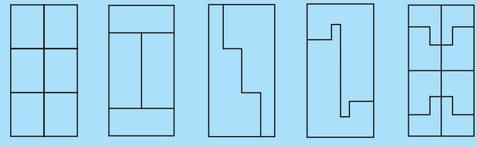The total area of rectangle

i) The first rectangle is divided into 6 equal parts. So the area of each part will be one-sixth of the total area

ii) The rectangle is divided into 4 equal parts, area of each part= one-forth area of rectangle= 6 cm2

iii) and (iv) are divided into two equal parts. Area of each part will be one half the total area of rectangle= 12  cm2

v) Area of rectangle is divided into 8 equal parts. Area of one part is one-eighth of the total area of rectangle = 3 cm2

## Solutions of NCERT for class 7 maths chapter 11 perimeter and area topic 11.3 area of a parallelogram

Question:1(i) Find the area of following parallelograms: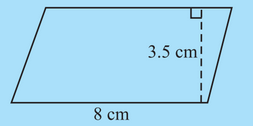Area of the parallelogram is the product of base and height

Question: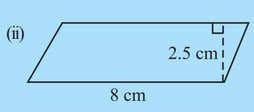Area of the parallelogram is the product of base and height

Question:(iii) Find the area of the following parallelograms:

In a parallelogram , and the perpendicular from  on is

Area of parallelogram = the product of base and height

## CBSE NCERT solutions for class 7 maths chapter 11 perimeter and area-Exercise: 11.2

Question:We know that

Area of parallelogram

Here,

Base of parallelogram = 7cm

and

Height of parallelogram = 4 cm

Therefore, the area of the parallelogram is

Question:We know that

Area of parallelogram

Here,

Base of parallelogram = 5cm

and

Height of parallelogram = 3 cm

Therefore, the area of the parallelogram is

Question: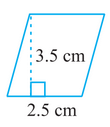We know that

Area of parallelogram

Here,

Base of parallelogram = 2.5cm

and

Height of parallelogram = 3.5 cm

Therefore, area of parallelogram is

Question: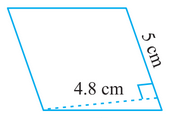We know that

Area of parallelogram

Here,

Base of parallelogram = 5cm

and

Height of parallelogram = 4.8 cm

Therefore, the area of a parallelogram is

Question: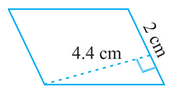We know that

Area of parallelogram

Here,

Base of parallelogram = 2 cm

and

Height of parallelogram = 4.4 cm

Therefore, area of parallelogram is

Question: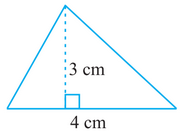We know that

Area of triangle

Here,

Base of triangle = 4 cm

and

Height of triangle =3 cm

Therefore, area of triangle is

Question: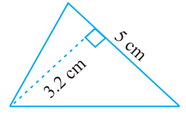We know that

Area of triangle

Here,

Base of triangle = 5 cm

and

Height of triangle =3.2 cm

Therefore, the area of the triangle is

Question: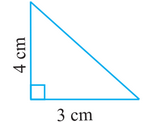We know that

Area of triangle

Here,

Base of triangle = 3 cm

and

Height of triangle =4 cm

Therefore, area of triangle is

Question: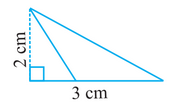We know that

Area of triangle

Here,

Base of triangle = 3 cm

and

Height of triangle =2 cm

Therefore, the area of the triangle is

Question:We know that

Area of parallelogram

a) Here, base and area of parallelogram is given

b) Here height and area of parallelogram is given

c) Here height and area of parallelogram is given

d) Here base and area of parallelogram is given

Question: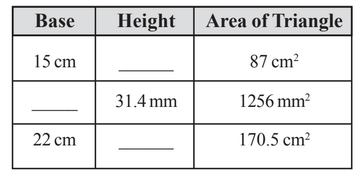We know that

Area of triangle

a) Here, the base and area of the triangle is given

b) Here height and area of the triangle is given

c) Here base and area of the triangle is given

Find:

We know that

Area of parallelogram

Here,

Base of parallelogram = 12 cm

and

Height of parallelogram = 7.6 cm

Therefore, area of parallelogram is

Find:

We know that

Area of parallelogram

Here,

Base of parallelogram = 12 cm

and

Height of parallelogram = 7.6 cm

Now,

Area is also given by

Therefore, value of QN is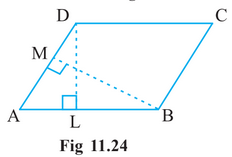We know that

Area of parallelogram

Here,

Base of parallelogram(AB) = 35 cm

and

Height of parallelogram(DL) = h cm

Similarly,

Area is also given by

Therefore, the value of BM and DL are  is  30cm and 42cm respectively

. Also find the length of.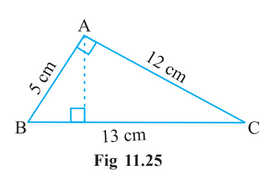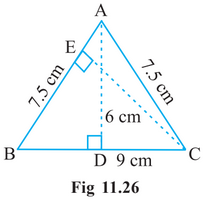We know that

Area of triangle

Now,

When base = 5 cm and height = 12 cm

Then, the area is equal to

Now,

When base = 13 cm and height = AD area remain same

Therefore,

Therefore, value of AD is    and the area is equal to

What will be the height from to i.e., ?We know that

Area of triangle

Now,

When base(BC) = 9 cm and height(AD) = 6 cm

Then, the area is equal to

Now,

When base(AB) = 7.5 cm and height(CE) = h , area remain same

Therefore,

Therefore, value of CE is  7.2cm  and area is equal to

## Question:(a)  In Fig Which square has a larger perimeter?

The outer square has a larger perimeter. Since each side of the inner square forms a triangle. The length of the third side of a triangle is less than the sum of the other two lengths.The arc length of the circle is slightly greater than the side length of the inner square. Therefore circumference of the inner circle is greater than the inner square

## NCERT solutions for class 7 maths chapter 11 perimeter and area-Exercise: 11.3

We know that

Circumference  of a circle is

Therefore, the circumference of the circle is  88 cm

We know that

Circumference  of circle is

Therefore, the circumference of the circle is  176 mm

We know that

Circumference of circle is

Therefore, circumference of circle is  132 cm

Question:

(Take )

We know that

Area of circle is

Therefore, the area of the circle is

Question:

We know that

Area of circle is

Therefore, area of circle is

Question:

We know that

Area of circle is

Therefore, the area of the circle is

It is given that  circumference of a circular sheet is  154 m

We know that

Circumference of circle is

Now,

Area of circle

Therefore, the radius and area of the circle are 24.5 m and   respectivelyIt is given that diameter of a circular garden is .

We know that

Circumference of circle is

Now, length of the rope requires to makes rounds of fence is

circumference of circle

Now,  cost of rope at  is

Therefore, length of the rope requires to makes rounds of fence is  132 m and   cost of rope at  is  Rs 528

We know that

Area of circle

Area of circular sheet with radius 4 cm

Area of the circular sheet with radius 3 cm

Now,

Area of remaining sheet = Area of circle with radius 4 cm - Area od circle with radius 3 cm

Therefore, Area of remaining sheet is

It is given that diameter of a circular table is 1.5m.

We know that

Circumference of circle is

Now, length of the lace required is

circumference of circle

Now,  cost of lace at  is

Therefore, length of the lace required is  4.71 m and   cost of lace at    is  Rs 70.65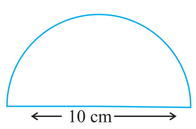It is given that the diameter of semi-circle is 10 cm.

We know that

Circumference of semi circle is

Circumference of semi-circle with diameter 10 cm  including diameter is

Therefore, Circumference of semi-circle with diameter 10 cm  including diameter is 25.7 cm

It is given that the diameter of a circular table is 1.6m.

We know that

Area of circle is

Now, the cost of polishing at  is

Therefore, the cost of polishing at     is  Rs 30.144

It is given that the length of wire is 44 cm

Now, we know that

Circumference of the circle (C) =

Now,

Area of circle (A) =

- (i)

Now,

Perimeter of square(P) =

Area of sqaure =

-(ii)

From equation (i) and (ii) we can clearly see that area of the circular-shaped wire is more than square-shaped wireIt is given that radius of circular card sheet is

Now, we know that

Area of circle (A) =

- (i)

Now,

Area of circle with radius 3.5 cm is

Area of two such circle is =                   -(ii)

Now, Area of rectangle =

-(iii)

Now, the remaining area is (i) - [(ii) + (iii)]

Therefore,  area of the remaining sheet  is

(Take  )

It is given that the radius of the circle is

Now, we know that

Area of the circle (A) =

- (i)

Now,

Now, Area of square  =

-(ii)

Now, the remaining area is (ii) - (i)

Therefore, the area of the remaining aluminium sheet  is

It is given that circumference of circle is

Now, we know that

Circumference of circle is =

Now, Area of circle (A) =

Therefore, radius and  area of the circle  are   respectively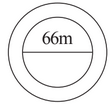It is given that the diameter of the flower bed is

Therefore,

Now, we know that

Area of the circle (A) =

-(i)

Now, Area of outer circle with radius(r ') = 33 + 4 = 37 cm is

-(ii)

Area of the path is equation (ii) - (i)

Therefore, the area of the path is

It is given that the radius of the sprinkler is

Now, we know that

Area of the circle (A) =

Area cover by sprinkle is

And the area of the flower garden is

Therefore,  YES  sprinkler water the entire garden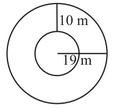We know that

Circumference of circle =

Now, the circumference of the inner circle  with radius (r) =  is

And the circumference of the outer circle  with radius (r ') = 19 m is

Therefore, the circumference of inner and outer circles are   respectively

It is given that radius of wheel is

Now, we know that

Circumference of circle =

Now, number of rotation done by wheel to go 352 m  is

Therefore,  number of rotation done by wheel to go 352 m is  200

It is given that minute hand of a circular clock is long  i.e. ( r = 15 cm)

Now, we know that one hour means a complete circle of minute hand

Now,

Circumference of circle =

Therefore,  distance cover by minute hand in one hour is  94.2 cm

Solutions of NCERT for class 7 maths chapter 11 perimeter and area topic 11.6 conversion of units

Question:(i) Convert the following:

1 cm = 10 mm

therefor

Question:(ii)

ha represents hectare

1 ha is 10000 m2

therefor

Question:(iii)

1m = 100cm

Therefor

Question:(iv) Convert the following:

The conversion is done as follows

## CBSE NCERT solutions for class 7 maths chapter 11 perimeter and area-Exercise: 11.4

It is clear that it is rectangular shaped with

We know that the area of the rectangle is

-(i)

Now, length and breadth of the outer rectangle isArea of the outer rectangle is

-(ii)

Area of the path is (ii) - (i)

Therefore, the area of the path is

It is given that park is   long and   broad

It is clear that it is rectangular shaped with

We know that area of rectangle is

-(i)

Now, length and breadth of outer rectangle isArea of outer rectangle is

-(ii)

Area of path is (ii) - (i)

Therefore,  area of path is

It is given that cardboard is  long and wide such that there is a margin of along each of its sides

It is clear that it is rectangular shaped with

We know that the area of the rectangle is

-(i)

Now, the length and breadth of cardboard without margin isArea of cardboard without margin is

-(ii)

Area of margin is (i) - (ii)

Therefore, the area of margin is

It is given that room  is long and  wide.

It is clear that it is rectangular shaped with

We know that the area of the rectangle is

-(i)

Now, when verandah of width   is constructed all along outside the room then length and breadth of the room  isArea of the room after verandah of width   is constructed

-(ii)

Area of verandah is (ii) - (i)

Therefore, the area of the verandah is

It is given that room  is long and  wide.

It is clear that it is rectangular shaped with

We know that the area of the rectangle is

-(i)

Now, when verandah of width   is constructed all along outside the room then length and breadth of the room  isArea of the room after verandah of width   is constructed

-(ii)

Area of verandah is (ii) - (i)

Therefore,  area of verandah is

Now, the cost of cementing the floor of the verandah at the rate of  is

Therefore,  cost of cementing the floor of the verandah at the rate of  is

Is is given that side of square garden is

We know that area of square is =

-(i)Now, area of square garden without 1 m boarder is

-(ii)

Area of path is (i) - (ii)

Therefore,  area of path is

Is is given that side of square garden is

We know that area of square is =

-(i)Now, area of square garden without 1 m boarder is

-(ii)

Area of path is (i) - (ii)

Therefore,  area of path is

Now,  cost of planting grass in the remaining portion of the garden at the rate of  is

Therefore,  cost of planting grass in the remaining portion of the garden at the rate of   isIt is given that width of each road is  and the length of rectangular park is  and breadth is

Now, We know that area of rectangle is =

Area of total park is

-(i)

Area of road parallell to width of the park ( ABCD ) is

-(ii)

Area of road parallel to length of park ( PQRS )  is

-(iii)

The common area of both the roads ( KMLN ) is

-(iv)

-(v)

Now, Area of the park excluding crossroads is =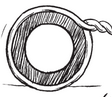It is given that the width of each road is  and length of rectangular park is  and breadth is

Now, We know that area of rectangle is =

Area of total park is

-(i)

Area of road parallell to width of the park ( ABCD ) is

-(ii)

Area of road parallel to length of park ( PQRS )  is

-(iii)

The common area of both the roads ( KMLN ) is

-(iv)

Therefore, the area of road isIt is given that the width of each road is  and the length of rectangular park is  and breadth is

Now, We know that area of rectangle is =

Area of the total park is

-(i)

Area of road parallel to the width of the park ( ABCD ) is

-(ii)

Area of road parallel to the length of park ( PQRS )  is

-(iii)

The common area of both the roads ( KMLN ) is

-(iv)

Now, the cost of constructing the roads at the rate of   is

Therefore, the cost of constructing the roads at the rate of   is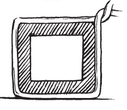It is given that the radius of the circle is 4 cm

We know that circumference of circle =

And perimeter of the square is

Length of cord left is

Therefore, Length of cord left is

We know that area rectangle is =

Area of rectangular land with length 10 m and width 5 m is

Therefore, area of rectangular land with length 10 m and width 5 m is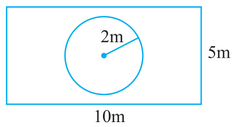We know that area of circle is =

Area of flower bed with radius 2 m is

Therefore, area of flower bed with radius 2 m isArea of the lawn excluding the area of the flower bed = Area of rectangular lawn - Area of flower bed

=

Therefore, area of the lawn excluding the area of the flower bed isWe know that circumference of the circle is =

Circumference of the flower bed with radius 2 m is

Therefore, the circumference of the flower bed with radius 2 m is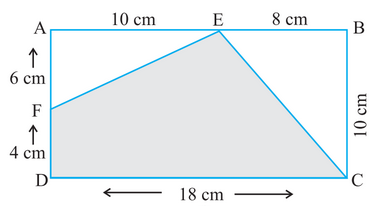Area  of shaded portion = Area of the whole rectangle ( ABCD ) - Area of two triangles ( AFE and BCE)

Area of the rectangle with length 18 cm and width 10 cm is

Area of triangle AFE with base 10 cm and height 6 cm is

Area of triangle BCE with base 8 cm and height 10 cm is

Now, Area  of the shaded portion is

Question: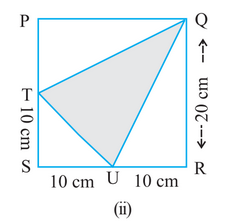Area  of shaded portion = Area of the whole square ( PQRS ) - Area of three triangles ( PTQ , STU and QUR )

Area of the square with side 20cm is

Area of triangle PTQ with base 10 cm and height 20 cm is

Area of triangle STU with base 10 cm and height 10 cm is

Area of triangle QUR with base 20 cm and height 10 cm is

Now, Area  of the shaded portion is

Question: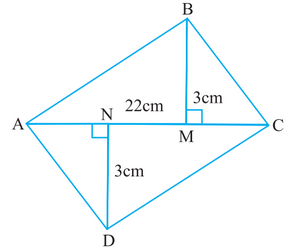Area  of  quadrilateral ABCD = Area of triangle ABC + Area of tringle ADC

Area of triangle ABC with base 22 cm and height 3 cm is

Area of triangle ADX with base 22 cm and height 3 cm is

Therefore, the area of quadrilateral ABCD is =

NCERT Solutions for Class 7 Maths- Chapter-wise

 Chapter No. Chapter Name Chapter 1 Solutions of NCERT for class 7 maths chapter 1 Integers Chapter 2 CBSE NCERT solutions for class 7 maths chapter 2 Fractions and Decimals Chapter 3 NCERT solutions for class 7 maths chapter 3 Data Handling Chapter 4 Solutions of NCERT for class 7 maths chapter 4 Simple Equations Chapter 5 CBSE NCERT solutions for class 7 maths chapter 5 Lines and Angles Chapter 6 NCERT solutions for class 7 maths chapter 6 The Triangle and its Properties Chapter 7 Solutions of NCERT for class 7 maths chapter 7 Congruence of Triangles Chapter 8 NCERT solutions for class 7 maths chapter 8 comparing quantities Chapter 9 CBSE NCERT solutions for class 7 maths chapter 9 Rational Numbers Chapter 10 NCERT solutions for class 7 maths chapter 10 Practical Geometry Chapter 11 Solutions of NCERT for class 7 maths chapter 11 Perimeter and Area Chapter 12 CBSE NCERT solutions for class 7 maths chapter 12 Algebraic Expressions Chapter 13 NCERT solutions for class 7 maths chapter 13 Exponents and Powers Chapter 14 Solutions of NCERT for class 7 maths chapter 14 Symmetry

## NCERT Solutions for Class 7- Subject-wise

Some important point from NCERT solutions for class 7 maths chapter 11 perimeter and area which you should remember-

Area of a triangle: If the base length and height of a triangle are given

Perimeter of the triangle:- Perimeter will be equal to the sum the sides of the triangle

a- First side of the triangle

b- Second side of the triangle

c- Third side of the triangle

Area of Circles:-

Circumference of Circle:-

Area of a parallelogram:b-> base length

h-> height

Tip- You shouldn't just memorize the formulas, also understand the concept of how these formulas are derived. If you go through solutions of NCERT for class 7 maths chapter 11 perimeter and area, you will understand all these concepts very easily.